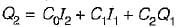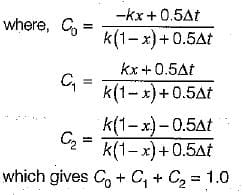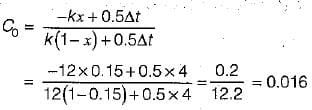# Test: Flood Routing - 1

## 10 Questions MCQ Test Topicwise Question Bank for Civil Engineering | Test: Flood Routing - 1

Description
Attempt Test: Flood Routing - 1 | 10 questions in 30 minutes | Mock test for Civil Engineering (CE) preparation | Free important questions MCQ to study Topicwise Question Bank for Civil Engineering for Civil Engineering (CE) Exam | Download free PDF with solutions
QUESTION: 1

Solution:
QUESTION: 2

Solution:
QUESTION: 3

### The St Venant equations for unsteady open-channel flow are

Solution:
QUESTION: 4

In routing a flood through a reach, the point of intersection of inflow and outflow hydrographs coincides with the peak of outflow hydrograph

Solution:
QUESTION: 5

Which of the following is a proper reservoir-routing equation?

Solution:
QUESTION: 6

The Muskingum method of flood routing is a

Solution:

In reservoir routing, the storage is a unique function of outflow discharge, S = f(Q).
However, in channel routing the storage is a function of both outflow and inflow discharge and hence a different routing method is needed from muskingum method of hydrological channel routing storage is given by,QUESTION: 7

The Muskingum method of flood routing assumes the storage S is related to inflow rate I and outflow rate Q of a reach as S =

Solution:

For a given channel reach by selecting a routing interval Δt and using the Muskingum equation, the change in storage is,
S2 - S1 = k(xI2 - I1) + 1 - x(Q2 - Q1)
Where suffixes 1 and 2 refer to the conditions before and after the time interval Δt.

QUESTION: 8

The Muskingum method of flood routing gives Q2 = C0I2 + C1I1 + C2Q1. The coefficients in this equation will have values such that

Solution:QUESTION: 9

The Muskingum channel routing equation is written for the outflow from the reach Q in terms of the inflow I and coefficients C0, C1 and C2 as

Solution:
QUESTION: 10

In the Muskingum method of channel routing the routing equation is written as Q2 = C0I2 + C1I1 + C2Q1. If the coefficients K = 12 h and x = 0.15 and the time step for routing Δt = 4 hr, the coefficient C0 is

Solution:Use Code STAYHOME200 and get INR 200 additional OFF Use Coupon Code